# Maths - Sets

A set is a collection of things, which are called the elements of the set.

"an abstract set X has elements each of which has no internal structure whatsoever" - Lawvere.

In mathematics 'set' is a fundamental concept but there are different approaches that can be taken to it:

• There are various formulations of set theory. In, say the ZF formulation, everything is a set.
• Often we may look at set as a sort of minimal structure onto which we can add extra structure.
• In computing a set may be seen as a collection, like a list, where there are no duplicates and order is not important.
• There is a correspondence between set theory and second order logic.

Here we mostly treat set as a minimal structure, with the concept of set membership and subsets, onto which we can add other structures.

## Concepts

 a ∈ A The basic concept of set theory is set membership. This expression means that 'a' is an element of 'A'.

Sets can be specified in various ways:

 {1,2,3} We can list all the elements, that is, build up the set from nothing: Ø {n | n<4} We can start with an existing set (in this case n - the set of natural numbers) and then apply constraints to it.

#### Principle of Comprehension

If φ(x) is a property of object x then there exists a set whose elements have that property.

## Equality of Types

 In Set Theory More on page here.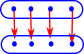Two sets are equal if they contain the same elements (Principle of Extensionality). If the set has structure then that would also have to be equal in some way. In Category Theory More on page here.Often when comparing types we need some loser way of considering that two types are essentially the same. Isomorphism is frequently the most appropriate type of equality. This is defined using a category theory way, that is, by external morphisms. In Type Theory More on page here.In Homotopy Type Theory there is a different way to get isomorphism from equality. That is to allow types to be equal in many ways.

#### Set Theory

In, say the ZF formulation of set theory, everything is a set.The elements of a set are themselves sets and their elements are sets. Its sets all the way down until we get to an empty set.

Also an element cannot occur more than once in a set so a given set can't contain more than one empty set.

An element can occur in more than one set but loops would allow paradoxes.

This is a difference between set theory and type theory, the page here discusses the relationship between set theory and type theory.## Set + Structure

In its purest form the only information contained in a set is the number of elements it contains. All other information, such as labeling the elements, can be added using functions between sets.

A total function maps every element in the domain to an element in the codomain. Although not every element in the codomain may necessarily be mapped too. So, in this way functions between sets are not necessarily symmetrical.

From this mathematical structure is genererated.

 Bijective functions are invertible. See: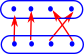Surjective functions are not invertible in that we can't reverse the function and get back to where we started, for instance if we have a surjective mapping from set to set we can't get back to the same set, however we can go back to a 'set of sets'. This gives rise to interesting structure, see:Injective functions gives a subobject structure. If we have an injective mapping from set to set we can't get back to the same set, however we can have a mapping from set to Bool which tells us which element are in the subset, this is known as a characteristic function in sets or 'subobject classifier' more generally in category theory. This subobject structure is interesting see: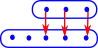A key part of mathematics is proofs.

In order to prove things about sets we need a logic layer underpinning it.

## Proofs in Isabelle

In Isabelle sets are typed. All elements have the same type (say τ) and the set itself has the type τ set (the order of type constructors is reversed compared to many languages). A type variable a is written: 'a so a set of these is written: 'a set.

Isabelle Notation Meaning
a ∈ A A is a member of B
a ∉ A A is not a member of B
{} empty set
UNIV universal set
{a,b,c} a finite set consisting of elements a,b and c
{x. P}

comprehension

set of all elements that satisfy predicate P

{p*q | p q. pprime /\ qprime}

more complicated comprehension in this case all numbers which are the products of two primes. Could also be represented by:

{x.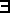p q. z = p*q /\ pprime /\ qprime}

AB A is a subset of B

### Set Membership

The Isabelle code (from this site) starts with two concepts:

• member () Membership is the key concept of sets. In Isabelle it is a binary operator which takes a element and a set and returns true if the element is a member of the set.
• Collect infers the members of a set from a predicate, this is known as set comprehension.
 ```axiomatization Collect :: "('a => bool) => 'a set" -- "comprehension" and member :: "'a => 'a set => bool" -- "membership" where mem_Collect_eq [iff, code_unfold]: "member a (Collect P) = P a" and Collect_mem_eq [simp]: "Collect (λx. member x A) = A" notation (HTML output) member ("op ∈") and member ("(_/ ∈ _)" [51, 51] 50) and not_member ("op ∉") and not_member ("(_/ ∉ _)" [51, 51] 50)```

The axioms show Collect and member as being some sort of inverse. that is, Collect allows us to compose a set and member allows us to decompose it.

In category theory a function a subobject classifier has the type: 'a set => bool.

Set comprehension is a construction that allows us to define a set by stating the properties of its members.

## Mappings

Mappings, functions between sets are discussed on page here.

## Subset

AB means A is a subset of B

AB iff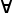xA : xB

#### Subsets and Logic

There is a relationship between subsets and logic.

This can give rise to different set theories, for instance, constructive or intuitionistic logic gives rise to constructive set theory.

## Intersection, Union and Venn Diagrams

### IntersectionThe intersection of two sets is another set which contains the elements in both the original sets.

### Union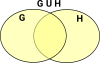The intersection of two sets is another set which contains all the elements from original sets, if an element is in both the original sets then it is only included once, not twice.

### ComplementThis is everything not in the set. This has to be defined in terms of the universe under discussion.

## Cartesian ProductThe Cartesian product of two sets is a set of pairs representing every combination of the two sets. This is a very general product that we can define regardless of whether multiplication is a valid operation for the elements of the sets being multiplied.

So this type of product 'generates' a higher dimensional quantity.

So what happens when we multiply two of these two dimensional quantities?

(g1,h1)(g2,h2) = ?

Perhaps it might generate a 4 dimensional quantity:

(g1,h1)(g2,h2) = (g1,h1,g2,h2)

Or we might be able to define a multiplication which would make this equivalent to complex number multiplication:

(g1,h1)(g2,h2) = (g1g2 - h1h2 , g1h2 + g2h1)

Or we may require a different definition,as an example, lets take the dihedral group D3, that we looked at on this page. We might represent any transform as a pair like:

(ma,ra)

Consisting of one reflection and one rotation, we can represent any element of this group by such a pair.

 Lets take another example, imagine that we have a group G and a subgroup H with elements g1,g2… and h1,h2…In this case we can now define multiplication as g1 H * g2 H = g1 g2 H

 g1 H g2 H g1 g2 Hleft coset g1 of H left coset g2 of H left coset g1,g2 of H

## ProductIf multiplying individual elements of the first set by elements of the second set is valid, then the product no longer needs to be represented by pairs, in this case the product contains the product of every combination of the two sets. If we are combining two groups then we may be able to use an external or internal product as described on this page.

## Equivalence Relation

Generalisation of equality to set theory.

If two sets are exactly equal, this may not be all that interesting from a mathematical point of view. It is often more interesting when two sets are not identical but preserve some form of structure when mapping between them.

type category notation
tighter Equality
Isomorphism 1 = GF
FG = 1
Equivalence 1GF
FG1
FG => 1

Equivalence:

Set Notation Alternative Notation Property
(a,a)R for all aS aa Reflective
(a,b)R implies (b,a)R ab implies ba Symmetric
(a,b)R and (b,c)R implies (a,c)R ab and bc implies ac Transitive

## Sequence in a set

A function from N (the set of positive integers) to the set.

In other words each element of the set is assigned a number.

Notation:

{an}n=1

## Indexing sets

An index set is a set used to label the elements of another set using a surjective function.An alternative approach is to attach a name to each element of a collection of sets.Notation:

{aα}α∈A

## Next

Mappings, functions between sets are discussed on page here.